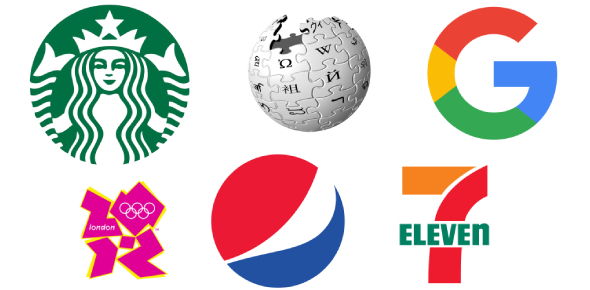# Complicated Exam On Science

15 Questions | Attempts: 162
ShareSettingsThis is a semi-complicated exam on science only 3 out of 20 students have passed

• 1.
What is the name for the steps scientists go through to solve problems?
• A.

A. drawing conclusions

• B.

B. forming a hypothesis

• C.

C. research design

• D.

D. scientific method

• 2.
How might a scientist show bias in an experiment?
• A.

A. by asking others to verify the data

• B.

B. by choosing a random sample by computer

• C.

C. by drawing conclusions without analyzing data

• D.

D. by using multiple trials to confirm data

• 3.
Mike is testing the effects of friction on a toy car going down a ramp. He runs one trial with sandpaper on the ramp and another trial with cloth on the ramp. What else does he need in his experiment?
• A.

A. a control group

• B.

B. a longer ramp

• C.

C. an independent variable

• D.

D. a technology

• 4.
Mike is conducting an experiment to see if his dog will eat a new brand of dog food. He will replace the dog’s old food with the new brand. Identify the dependent variable in this experiment.
• A.

A. the amount of food

• B.

B. the place the food is left

• C.

C. the type of food

• D.

D. whether the dog eats

• 5.
Why is it important for scientists to present their findings to the public?
• A.

A. The public has to understand every scientific finding.

• B.

B. Scientific data can benefit society in different ways.

• C.

C. The scientist might be able to get the data published.

• D.

D. The scientist needs credit for his or her findings.

• 6.
Clara is conducting an experiment to find out the number of times her lab mice received a reward for finishing a task. She is using a tally chart to keep track of each mouse and the number of rewards each receives. What is the best type of graph for her to use to analyze her data?
• A.

A. bar graph

• B.

B. line graph

• C.

C. pictograph

• D.

D. pie graph

• 7.
Scientists use prior experience to ____.
• A.

A. collect data

• B.

B. make predictions about what will happen under certain circumstances

• C.

C. analyze their data

• D.

D. serve as a control in their experiments

• 8.
After scientists analyze the results of their experiments, they ____.
• A.

A. form hypotheses

• B.

B. include a control

• C.

C. communicate those results to other people

• D.

D. choose the variables they want to test

• 9.
Which of the following steps to solve a problem must be completed first?
• A.

A. analyzing data

• B.

B. recognizing and identifying the problem

• C.

c. forming a hypothesis

• D.

D. testing a hypothesis

• 10.
Which of the following steps to solve a problem is completed last?
• A.

A. analyzing data

• B.

B. recognizing and identifying the problem

• C.

C. drawing conclusions

• D.

D. testing a hypothesis

• 11.
A prediction or statement that can be tested is ____.
• A.

A. a conclusion

• B.

B. an observation

• C.

C. a control

• D.

D. a hypothesis

• 12.
A factor in an experiment that can change is ____.
• A.

A. an observation

• B.

B. a variable

• C.

C. a control

• D.

D. a hypothesis

• 13.
A sample that is treated exactly like the other experimental groups except that the variable is not applied to it is a(n) ____.
• A.

A. observation

• B.

B. variable

• C.

C. control

• D.

D. hypothesis

• 14.
Scientists conduct multiple trials of their experiments because ____.
• A.

A. their results are more likely to be reliable

• B.

B. it costs less to run more trials than fewer trials

• C.

C. unusual occurrences are likely to be repeated every time

• D.

D. experiments always have the same results

• 15.
A good way to organize and record your results and observations is ____.
• A.

A. in a data table

• B.

B. by using a calculator

• C.

C. with a balance or spring scale

• D.

D. by having a hypothesis before you begin your experiment

## Related TopicsBack to top
×

Wait!
Here's an interesting quiz for you.